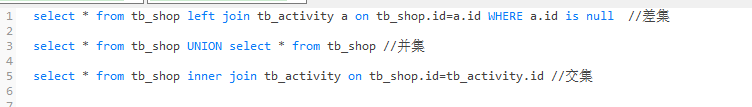• Latex交集并集
2021-06-11 13:19:26

(持续整理，为了防止偷懒，我决定只要一用到，我就来这里增加)

并集: A ∪ B A\cup B

$A\cup B$


交集: A ∩ B A\cap B

$A\cap B$latex
更多相关内容
• 利用带头结点的单链表实现两个集合的并、交、差运算。（难易程度：低） [实验目的] 1、掌握线性表的链表存储结构。 2、掌握在单链表上基本操作的实现。...4、要求不改变原来的集合，并集交集和差集分别另外存放。c语言
• matlab 中连续区间进行交并集操作，输入输出为向量表示的连续区间如A=[a,b,c,d]表示A=（a,b）U(c,d)，A为最简表达式，各个集合不相交matlab
• 主要介绍了pandas DataFrame 交集并集补集的实现，文中通过示例代码介绍的非常详细，对大家的学习或者工作具有一定的参考学习价值，需要的朋友们下面随着小编来一起学习学习吧
• ①差集 方法一： if __name__ == '__main__': a_list = [{'a' : 1}, {'b' : 2}, {'c' : 3}, {'d' : 4}, {'e' : 5}] b_list = [{'a' : 1}, {'b' : 2}] ret_list = [] for item in a_list: if item not in b_...
• ## 交集并集差集补集

千次阅读 2021-08-23 20:22:24
对于两个给定集合A、B，由两个集合所有元素构成的集合，叫做AB的并集。 记作：AUB 读作“A并B” 例：{3,5}U{2,3,4,6}= {2,3,4,5,6} 2. 交集 对于两个给定集合A、B，由属于A又属于B的所有元素构成的集合，叫做AB...1. 并集
对于两个给定集合A、B，由两个集合所有元素构成的集合，叫做A和B的并集。
记作：AUB 读作“A并B”
例：{3,5}U{2,3,4,6}= {2,3,4,5,6}

2. 交集
对于两个给定集合A、B，由属于A又属于B的所有元素构成的集合，叫做A和B的交集。
记作： A∩B 读作“A交B”
例：A={1,2,3,4,5}，B={3,4,5,6,8}，A∩B={3,4,5}

3. 差集
记A，B是两个集合，则所有属于A且不属于B的元素构成的集合，叫做集合A减集合B(或集合A与集合B之差)，类似地，对于集合A、B，把集合{x∣x∈A,且x∉B}叫做A与B的差集。
记作：B-A

4. 补集
一般地，设S是一个集合，A是S的一个子集，由S中所有不属于A的元素组成的集合，叫做子集A在S中的绝对补集。
记作：∁UA，包括三层含义：
1）A是U的一个子集，即A⊊U;
2）∁UA表示一个集合，且∁UA⊊U;
3）∁UA是由U中所有不属于A的元素组成的集合，∁UA与A没有公共元素，U中的元素分布在这两个集合中。

总结：

• 交集：A & B，即A与B ( x x　( 😊 😊 ) x　x　)
• 并集：A | B， 即A或B ( 😊 😊 ( 😊 😊 ) 😊 😊 )
• 差集：A - B， 即A减B ( 😊 😊 ( x　x )　x　x　）
• 补集：A ^ B，即A异B ( 😊 😊 ( x x )　😊 😊　）

交集和差集互反，即交差互补

展开全文• 主要介绍了Python实现两个list求交集并集，差集的方法,结合实例形式分析了Python使用intersection、union及difference方法实现两个集合list的交集并集与差集操作技巧,需要的朋友可以参考下
• 首先是数组合并，关于array_merge使用‘+’运算，可以参考之前的一篇文章。 https://blog.csdn.net/teng_liang/article/details/111937963 数组取交集 array_intersect() 第一个数组是主值，返回有相同元素的值...

PHP开发过程中，经常会对数组做出处理，在这里对数组的合并，交集，差集做了整理。

## 首先是数组合并，关于array_merge和使用‘+’运算，可以参考之前的一篇文章。

https://blog.csdn.net/teng_liang/article/details/111937963


## 数组取交集 array_intersect()

第一个数组是主值，返回有相同元素的值（判断依据是元素字符串相同，即 (string) $elem1 === (string)$elem2），键值保留第一个元素的键值

$array1 = array("a" => "green", "red", "blue");$array2 = array("b" => "green", "yellow", "red");
$result = array_intersect($array1, $array2); print_r($result);


结果

Array ( [a] => green  => red )


### array_intersect_assoc()

array_intersect_assoc不仅检查元素值，还会检查键名，都相同的元素才会保留下来，键值对 key => value 中的两个值仅在 (string) $elem1 === (string)$elem2 时被认为相等

$array1 = array("a" => "green", "b" => "brown", "c" => "blue", "red", 100 => 'a100');$array2 = array("a" => "green", "b" => "yellow", "blue", "red", '100' => 'a100');
$result_array = array_intersect_assoc($array1, $array2); print_r($result_array);


结果

Array ( [a] => green  => a100 )


## 差集 array_diff()

返回前面数组有而后面没有的元素数组，保留前面元素的键名，相同判断是根据字符串值相同，即 (string) $elem1 === (string)$elem2

$array1 = array("a" => "green", "red", "blue", "red");$array2 = array("b" => "green", "yellow", "red");
$result = array_diff($array1, $array2); print_r($result);


结果

Array (  => blue )


### array_diff_assoc()

array_diff_assoc不仅检查元素，还检查键名，键值对 key => value 中的两个值仅在 (string) $elem1 === (string)$elem2 时被认为相等

$array1 = array("a" => "green", "b" => "brown", "c" => "blue", "red");$array2 = array("a" => "green", "yellow", "red");
$result = array_diff_assoc($array1, $array2); print_r($result);


结果

Array ( [b] => brown [c] => blue  => red )


在来个例子

$array1 = array(0, 1, 2);$array2 = array("00", "01", "2");
$result = array_diff_assoc($array1, $array2); print_r($result);


结果

Array (  => 0  => 1 )

展开全文php
• 今天小编就为大家分享一篇pandas进行数据的交集并集方式的数据合并方法，具有很好的参考价值，希望对大家有所帮助。一起跟随小编过来看看吧
• MySQL求交集 并集 差集 交集 两个表之间相同的 并集 两个表之间的总和 ps: union 自带去重 差集 两个表直接的差值MySQL求交集 并集 差集

交集 两个表之间相同的

并集 两个表之间的总和

ps: union 自带去重

差集 两个表直接的差值

展开全文mysql
• 主要介绍了python获得两个数组交集并集、差集的方法,实例分析了Python操作list集合的技巧,具有一定参考借鉴价值,需要的朋友可以参考下
• const arr1 = [1,2,3,4,5], arr2 = [5,6,7,8,9], ...// 交集 let intersection = arr1.filter(item => _arr2Set.has(item)) // 并集 let union = Array.from(new Set([...arr1, ...arr2])) // 补集 两个数组...javascript
• 有什么不足之处欢迎评论，作者水平不高！ #include<stdio.h> #include<stdlib.h> typedef struct LNode{ int date;//数据域 struct LNode *next; //指针域 }LNode,*LinkList;...void InitLinkL...单链表
• 一、交集和并集 1.1交集 两个或者多个集合之间用&符号链接实现，提取共有元素 1.2并集 两个或者多个集合之间用 | 符号连接实现，提取所有元素 二、公共方法 2.1 + 合并意思（字符串，列表，元组） ...python
• #set 函数是无序不重复的元素集合，可以进行关系测试，去除重复数据，还可以计算交集、差集、和并集 #class set（[iterable]） iterable 可迭代对象 #删除重复 x=set('sixbobo') y=set('googlebb') print('删除重复...
• 注意：是retainAll 不是什么contains 并集： 不去重：list1.addAll(list2); 去重：思路是:先将list中与list2重复的去掉，之后将list2的元素全部添加进去。 public static void test1(List list1, List list2) { ...
• 并集：array_merge（） 交集：array_intersect（） 差集：array_diff() 过滤：array_filter() 去重：array_unique()
• 目录----------前言------------交集和并集的符号巧记基本初等函数 ----------前言------------ 因为高中数学没学好，带有些主观看法 总是记忆混淆 交集和并集，最近在准备升本考试，这些逃不过去了，从容面对一下，...算法
• 下面小编就为大家分享一篇java8 集合求差集、并集交集的实例，具有很好的参考价值，希望对大家有所帮助。一起跟随小编过来看看吧
• 交集运算符 空格，可以是 EXCEL交集运算符 =G16:G18 G18:G20=G18得内容 如果两者没有交集会返回空！ SUM(G10:G12 I10:I12) =#NULL!或 G10:G12 I10:I12=#NULL!...并集呢 也不能用VBA的 union()excel vba
• 集合这种数据类型我们数学中所学的集合很是相似，数学中堆积的操作也有交集并集和差集操作，python集合也是一样。python 后端
• Python 中对 list 进行交集并集，差集操作，需要先将 list 转换为 set，再进行操作 交集 同时存在于A 与 B 中的元素 A = [1,2,3,4] B = [2,3,4,5] list(set(A) & set(B)) list(set(A).intersection(set(B))) ...python
• 交集 交集的方法:retainAll 取两个集合中包含相同的对象或者元素 例 有两个newCouresoldCourses可以使用retainAll方法oldCourses.retainAll(newCoures) 如果有相同的元素oldCourses中进保留相同的元素 如果不...
• C++ stl set 求集合的交集并集差集 编译环境为dev C++
• 算法流程： 从数组1的尚未比较的元素中拿出第一个元素array1(i)，用array1(i)与array2(j)进行比较（其中j>i且j的长度），可能出现下面两种情况， 1． 数组2中找到了一个与array1(i)相等的元素，则将array2(j)与array......